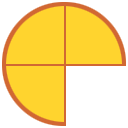# Common fraction

A common fraction is a fraction in which the numerator and denominator are integers. They are also sometimes referred to as vulgar, or simple fractions. Common fractions are typically expressed as either proper or improper fractions.Proper fractions are those with values less than 1 while improper fractions are those with values greater than (or equal to) 1. In both types of fractions, the numerator and denominator must be integers to be considered common fractions.

## Uses of common fractions

Common fractions can be used to represent a number of different things, such as a part of a whole, division, and a ratio.

### Part of a whole or set

A common fraction can represents part of a whole or a set. In the figure below, the pie can be represented by the common fraction ¾. There are 4 slices in the whole pie, but since one is missing, there are a total of 3 parts of the whole pie left, or ¾.### Division

Common fractions can be read as a division problem where the numerator is the dividend, the denominator is the divisor, and the fraction bar is the division symbol.

Example

Nancy wants to make omelets for breakfast. She has 6 eggs and she needs 2 eggs for each omelet. How many omelets can she make?Thus, Nancy can make 3 omelets.

### Ratio

A common fraction can be written in the form of a ratio.

Examples

1. Dante's birthday party included party hats and noisemakers for each guest. For each guest, there was one hat and two noisemakers. Express the ratio of party hats to noisemakers as both a common fraction and a ratio.

The ratio of hats to noisemakers is 1:2, or ½.

2. In the figure below, there are 4 bees and 1 hive. The ratio of bees to hives can therefore be expressed as the common fraction, or as the ratio 4:1.